# Perpendicular

What is the slope of the perpendicular bisector of line segment AB if A[9,9] and B[9,-2]?

k =  0

### Step-by-step explanation: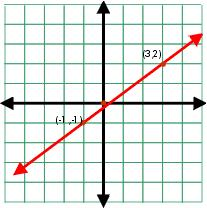Did you find an error or inaccuracy? Feel free to write us. Thank you!Tips to related online calculators
Line slope calculator is helpful for basic calculations in analytic geometry. The coordinates of two points in the plane calculate slope, normal and parametric line equation(s), slope, directional angle, direction vector, the length of the segment, intersections of the coordinate axes, etc.

## Related math problems and questions:

• Perpendicular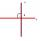Determine the slope of the line perpendicular to the line p: y = -x +4.
• Slope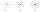Calculate the slope of a line that intersects points (-84,41) and (-76,-32).
• V - slopeThe slope of the line whose equation is -3x -9 = 0 is
• SlopeWhat is the slope of a line with an inclination 6.06 rad?
• Slope formFind the equation of a line given the point X(8, 1) and slope -2.8. Arrange your answer in the form y = ax + b, where a, b are the constants.
• Line segmentFor the line segment whose endpoints are L[-1, 13] and M[18, 2], find the x and y value for the point located 4 over 7 the distance from L to M.
• Slope RR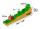A line has a rise of 2 and a run of 11. What is the slope?
• Midpoint of segmentPoint A has coordinates [4; -11] and the midpoint of the segment AB is the point [17; -7]. What are the coordinates of point B?
• SlopeFind the slope of the line: x=t and y=1+t.
• Right triangle from axesA line segment has its ends on the coordinate axes and forms with them a triangle of area equal to 36 square units. The segment passes through the point ( 5,2). What is the slope of the line segment?
• AngleA straight line p given by the equation y = (-8)/(6) x +78. Calculate the size of angle in degrees between line p and y-axis.
• Points on line segmentPoints P & Q belong to segment AB. If AB=a, AP = 2PQ = 2QB, find the distance: between point A and the midpoint of the segment QB.
• Cone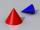If the segment of the line y = -3x +4, that lies in quadrant I, is rotated about the y-axis, a cone is formed. What is the volume of the cone?
• General line equationsIn all examples, write the GENERAL EQUATION OF a line that is given in some way. A) the line is given parametrically: x = - 4 + 2p, y = 2 - 3p B) the line is given by the slope form: y = 3x - 1 C) the line is given by two points: A [3; -3], B [-5; 2] D) t
• Three points 2The three points A(3, 8), B(6, 2) and C(10, 2). The point D is such that the line DA is perpendicular to AB, and DC is parallel to AB. Calculate the coordinates of D.
• Midpoint 4If the midpoint of a segment is (6,3) and the other endpoint is (8,-4), what is the coordinate of the other end?
• SlopeWhat is the slope of the line defined by the equation -2x +3y = -1 ?